Switches

Switches or buttons are electronic components that disconnect or connect two nodes of a circuit. In a physical realization of a circuit, these words may may refer to mechanical switches or buttons, but also apply to more complex active components that perform a similar action, such as transistor-based switches. They can also be considered as purely theoretical constructs in analyzing a circuit.

A switch has two states: either the two nodes can be connected or disconnected.

In an ideal switch, the connected state behaves like a resistor $R=0$ (short circuit), while the disconnected state behaves like a resistor $R=\infty$ (open circuit):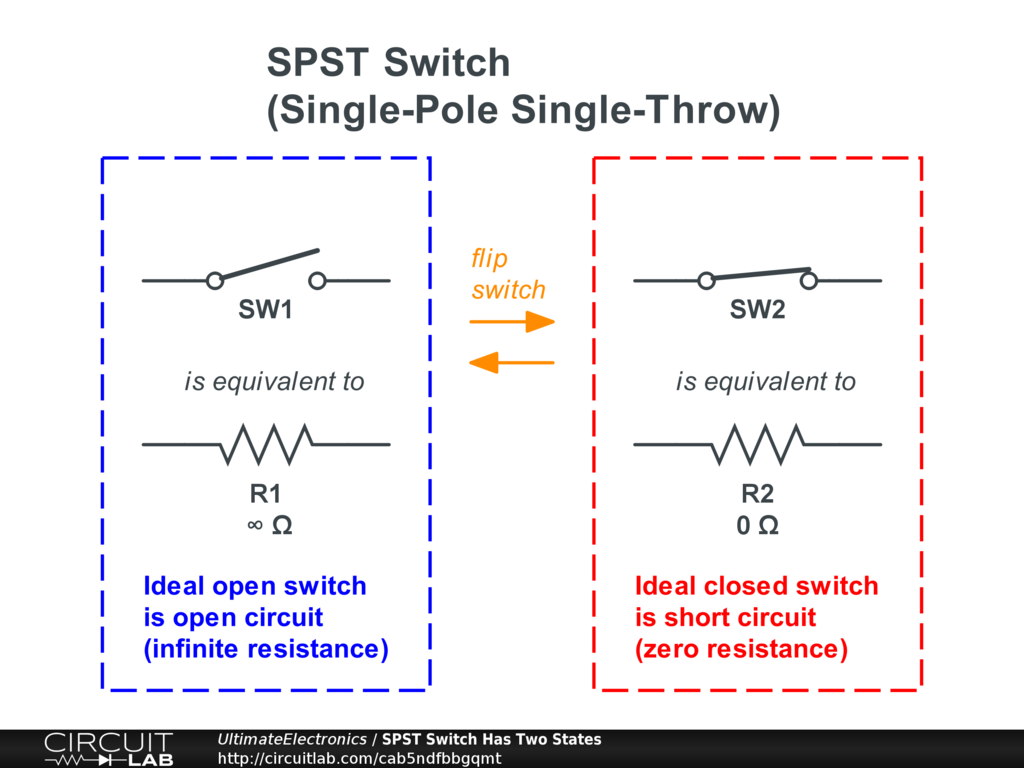The switch shown above is a "single throw" switch, meaning the switchable terminal can either be connected or not. Overall, this is called a SPST switch, for Single-Pole Single-Throw. This is useful as a simple on-off switch, like most light switches you're familiar with.

Multiple Throw

Another variation is double-throw, where instead of being disconnected, the switch becomes connected to some third node. In one state, nodes P and A are connected together, while B is disconnected. In the other state, nodes P and B are connected together, while A is disconnected. This is called a SPDT switch, for Single-Pole Double-Throw: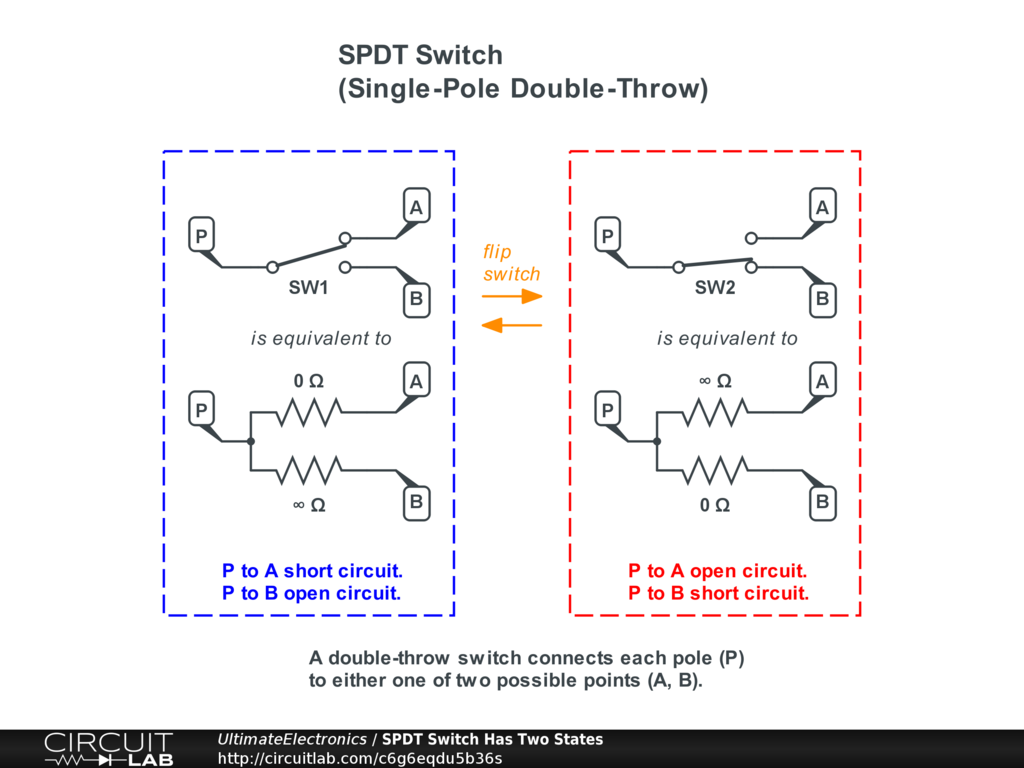An SPDT switch can be useful to connect one terminal to two mutually exclusive alternatives. For example, we can switch between two different amplifier input channels using an SPDT switch.

Switches can also be manufactured with more than two connection options, such as a rotary dial that can be set to one of 10 different positions. These are generally called "positions" instead of "throw," but the concept is the same.

Practical Switches

Practical switches differ from ideal switches in a number of important ways.

Real switches have some finite, nonzero resistance when closed. This resistance causes a voltage drop which may or may not be significant depending on the rest of the circuit. Usually, we wish that the on-resistance of the switch is much less than the resistance of whatever it's connected to so that we can approximate it as zero, but in practical design we may have to account for the finite resistance of a switch in higher-current situations. See Resistors in Series & Parallel and Algebraic Approximations for more.

Real switches have current limits because their nonzero resistance causes them to overheat in the on (closed) state. See Practical Resistors: Power Rating (Wattage) for more.

Real switches have voltage limits for their off (open) state. A high voltage between nearby components inside the switch creates a large electric field, which if high enough, could cause an arc or spark, where the electric field is strong enough that electrons jump through the air between the two terminals. This is definitely undesired and can damage the switch and whatever it's connected to by oxidizing and corroding the contacts, which leads to higher resistance in the future, and eventually more switch heating and possible failure.

Real switches can also have physical behavior like "bouncing" or "chattering," which is some rapid switching between on and off states in the milliseconds after a switch is closed. This is due to the mechanical springiness of the switch, and just like a car going over a speed bump, the contact may bounce a bit before it settles down to make steady contact. If you have access to an oscilloscope, you can see this phenomenon fairly easily. This leads to a set of "debouncing" techniques, including both using capacitor and software solutions to filter out these transient open-close cycles. If we didn't have debouncing, then each time you pressed a key on your computer keyboard, it might type that character a dozen times, instead of just once!

Real switches tend to degrade over time. Every time a switch or button is pressed, there are motions which can eventually cause materials to deform, either completely or just enough that there isn't as much pressure on the switch contacts. Additionally, switch contacts corrode over time depending on their materials and the environment they're exposed to, which can prevent them from making a good connection.

Real multiple-throw switches such as the SPDT switches shown above won't switch states instantaneously. Some are "make-before-break," in that they will momentarily be connected to both terminals while in transit. Others are "break-before-make," where the switched terminal will momentarily be connected to nothing at all. Either one can be bad depending on your use case. If you're working with a real switch or button and don't know which it is, it could be either: when possible, design your circuit not to break in either case.

Momentary Buttons vs. Toggle Switches

Electrically speaking, there is no real difference between a button and a switch. Mechanically, however, there is: a switch is mechanically toggled to stay open or stay closed, after which it stays in that position. In contrast, a button has a spring so that once the applied mechanical force is released, the button automatically reverts to its "normal" state.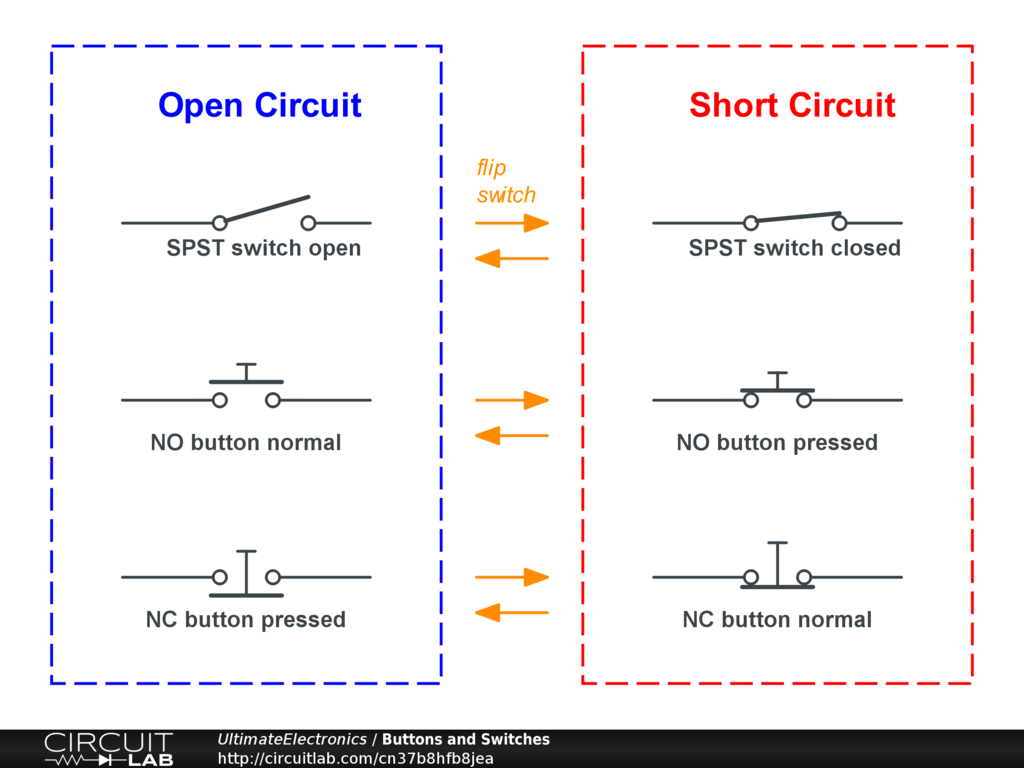Buttons are categorized as "normally open" or "normally closed." A normally open (NO) button is open circuit until pressed. A normally closed (NC) button is closed until pressed.

Both types of buttons are useful. A car's steering wheel may include a normally open button to activate the horn, allowing current to the horn only when it's being pressed. An electromagnetic door lock system may use a normally closed button, so that pressing the button cuts the current to the lock so the door can be opened.

In fact, the two can be usefully combined: the door latch mechanism of every microwave oven includes two NO buttons and one NC button. The three act together as a safety interlock to make sure the microwave oven's high power magnetron can't be turned on unless the door is really closed. The normally open buttons must both indicate that they're being pressed by the top and bottom latches of the door. The normally closed button is an extra safety backup, intentionally designed to blow a fuse rather than let the magnetron operate (unless the door is closed and the NC button becomes open).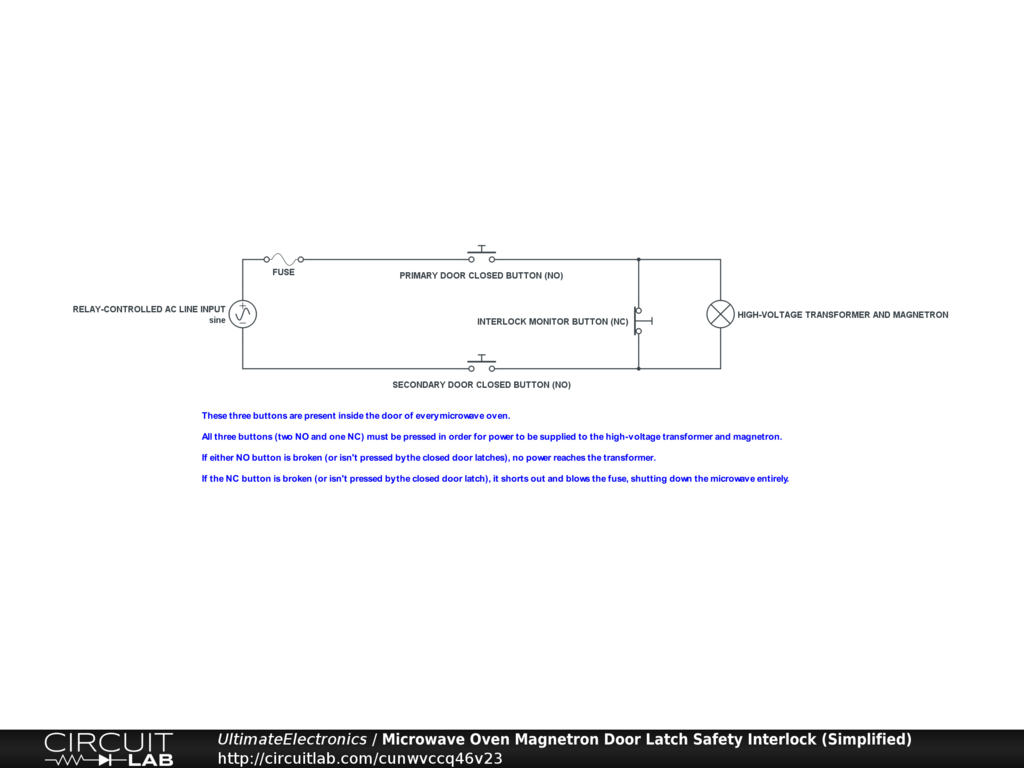Interactive Exercise Click to open the circuit above and inspect the configuration of buttons. All three buttons must be pressed by various parts of the door latch in order for the microwave oven to start cooking.

Multiple Poles

It's often useful to have one physical switch or button physically actuate multiple electrical switches. Instead of "single pole," these are called multiple pole switches, such as DPST (Double-Pole Single-Throw) and DPDT (Double-Pole Double-Throw).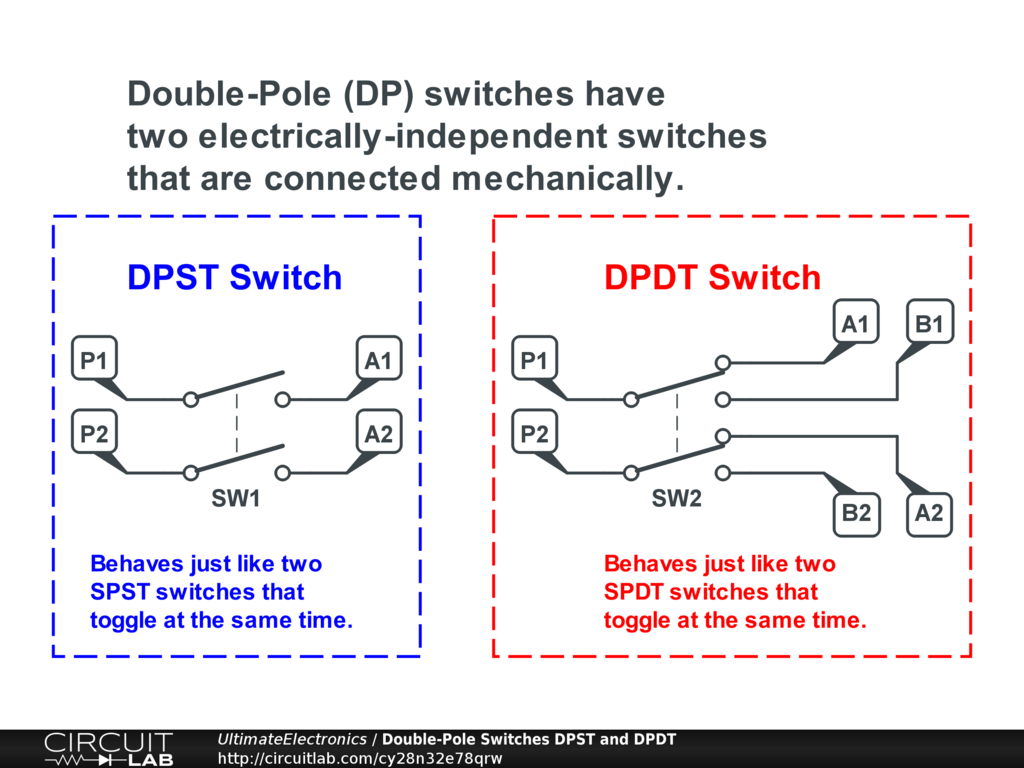These simply represent electrically-independent switches for each of the poles; there is no electrical connection between them, but there is a mechanical one. This means they may not switch at exactly the same time, and they may have different electrical properties such as corrosion on one but not the other.

Multiple-pole switches are useful in many situations, like switching both the left and right channels of an audio signal. Another example would be a power supply switch for a circuit that requires two different power input voltages (such as $\pm 12 \ \text{V}$):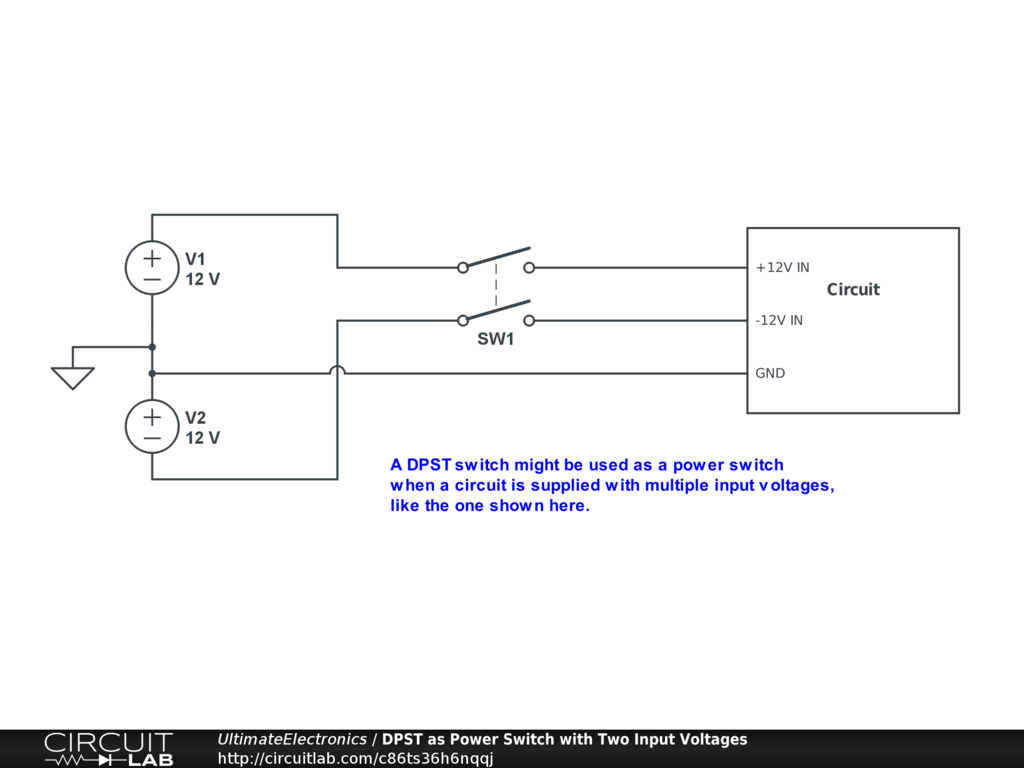In this example, however, we'd want to be careful to think about all the possible edge cases that can happen: what if one of the two internal SPST switches gets corroded, but not the other one? What if one simply makes contact a millisecond before the other? These could be important issues to consider depending on the circuit under consideration.

Solving Circuits with Switches

Any time we have a switch in a circuit, we have effectively multiple different circuits to solve independently.

For a circuit with a single two-posistion switch (including any SPST, SPDT, DPST, or DPDT), there are now two possible states of the circuit, each of which has its own solution.

In general, for a circuit with $N$ different two-position switches, the circuit solution becomes split into $2^N$ different configurations, each with its own solution. (If any switches have more than two possible positions, then we multiply by that instead of the number 2.)

This may seem like an enormously complicated problem, and in fact, it is! For example, if we think about about a single house or apartment as a single circuit, and consider all the light switches and all the power switches on all the devices plugged in, then there's quickly a huge number of possible configurations. If there are just 10 switches, there are already $2^{10} = 1024$ configurations with possibly different solutions. But this is reality: turning on the power switch for a blow dryer in the bathroom may make the lights dim in the kitchen. In practice, though, we often want to design circuits so that many variables are approximately independent of others, which we'll discuss more in the Voltage Dividers, Current Dividers, and Approximations section. However, in general, interactions do happen, and we have to re-solve because it's possible for all of the currents and voltages to change any time a switch is flipped.

Resistive Circuit with an SPST Switch

Here's a simple resistive circuit with a single SPST switch inside, labeled SW1: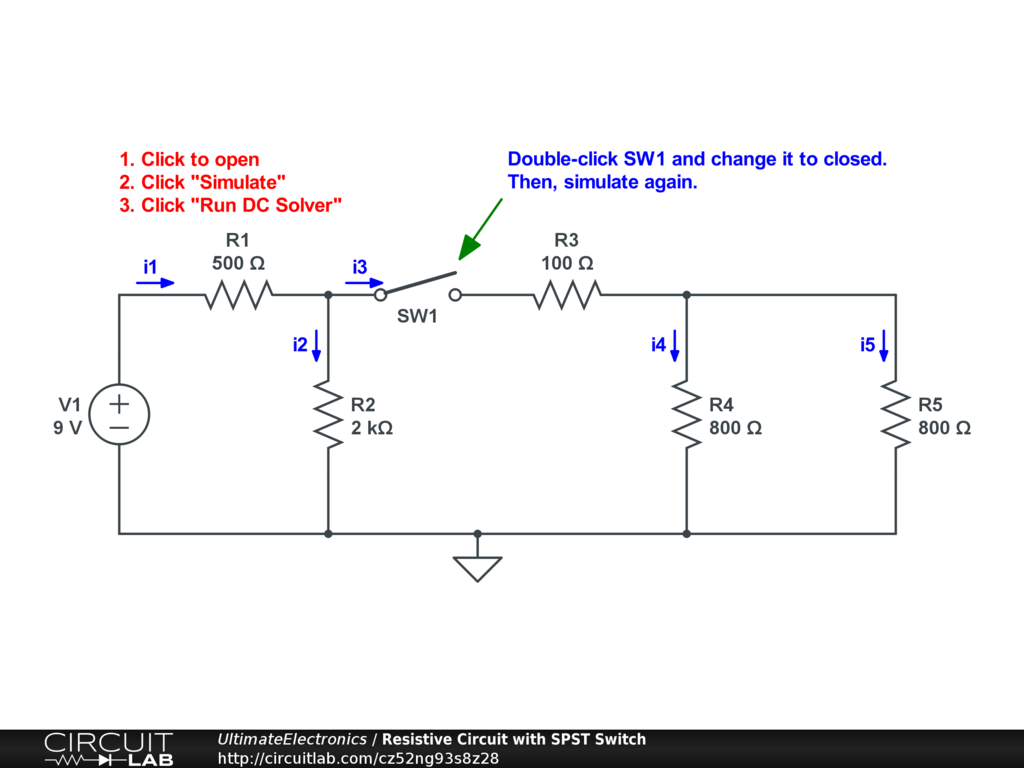Interactive Exercise Click the circuit, run the DC solver, and look at the currents in the circuit. With SW1 open, there's effectively no current through $i_3, i_4, i_5$.

Note that because of the way circuit simulators simulate open switches as very high but not infinite resistances, the current may not be exactly zero, but will be extremely small, perhaps a few femtoamps that "leaks" through the switch. (See Orders of Magnitude, Logarithmic Scales, and Decibels.)

Now, double click on the switch SW1, and set it to be closed. Re-run the DC solver. What are the currents now? In this case, most of the current does flow through branches $i_3, i_4, i_5$.

We can solve the system by hand by considering the two cases separately: SW1 open, and SW1 closed.

With SW1 Open

With the switch open, we can remove the switch entirely, because an open switch is open circuit ($R = \infty$):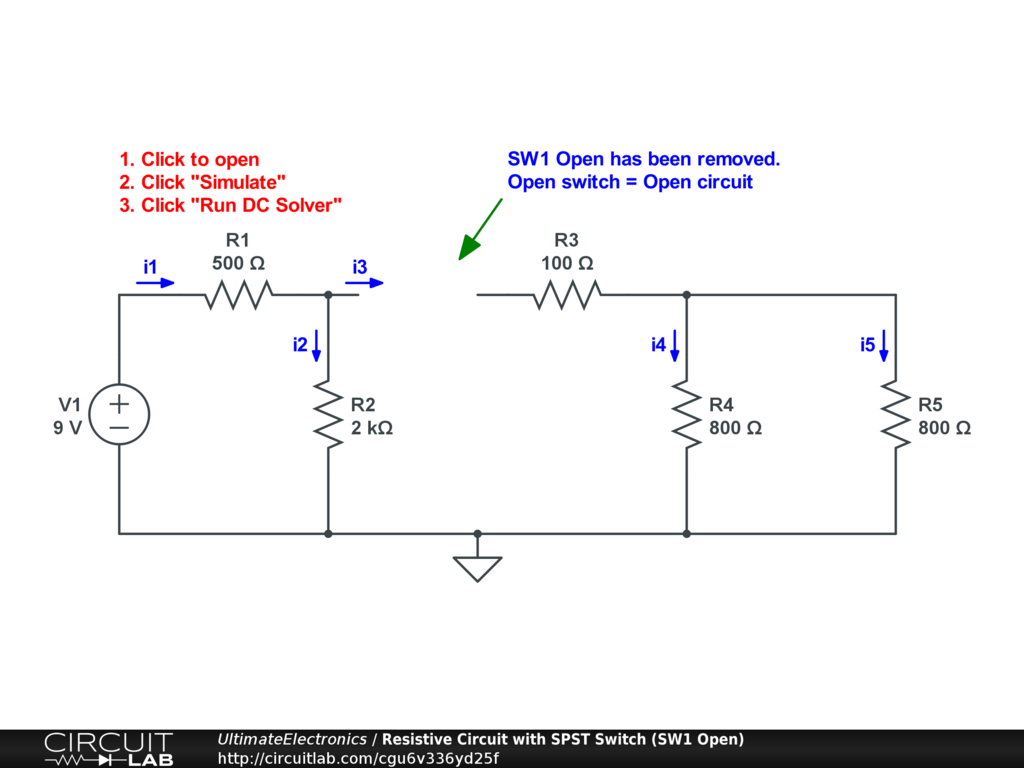From this schematic, it's clear that there is nothing to power resistors R3, R4, and R5, so all their branch currents are zero:

$$i_3 = i_4 = i_5 = 0$$

R1 and R2 are simply resistors in series, so we can add their resistances to find an effective resistance:

$$R_{\text{eq}} = R_1 + R_2 = 2500 \ \Omega$$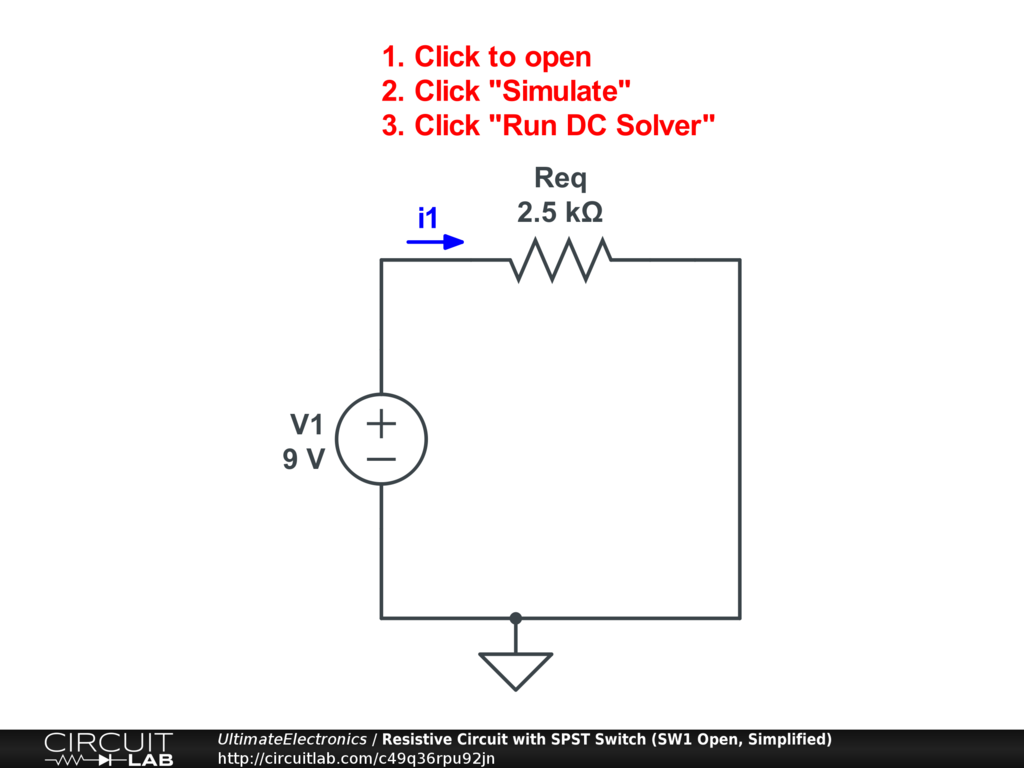Now, we can use Ohm's Law to find the total current:

$$I = \frac {V} {R_{\text{eq}}} = \frac {9} {2500} = 0.0036 = 3.6 \ \text{mA}$$

Because of Kirchhoff's Current Law, the branch currents $i_1 = i_2$, so:

$$i_1 = i_2 = 3.6 \ \text{mA}$$

We've fully solved for all the currents with the switch open, and it would now be easy to find the node voltages as well.

With SW1 Closed

With the switch closed, we can replace it with a wire, because a closed switch is short circuit ($R = 0$):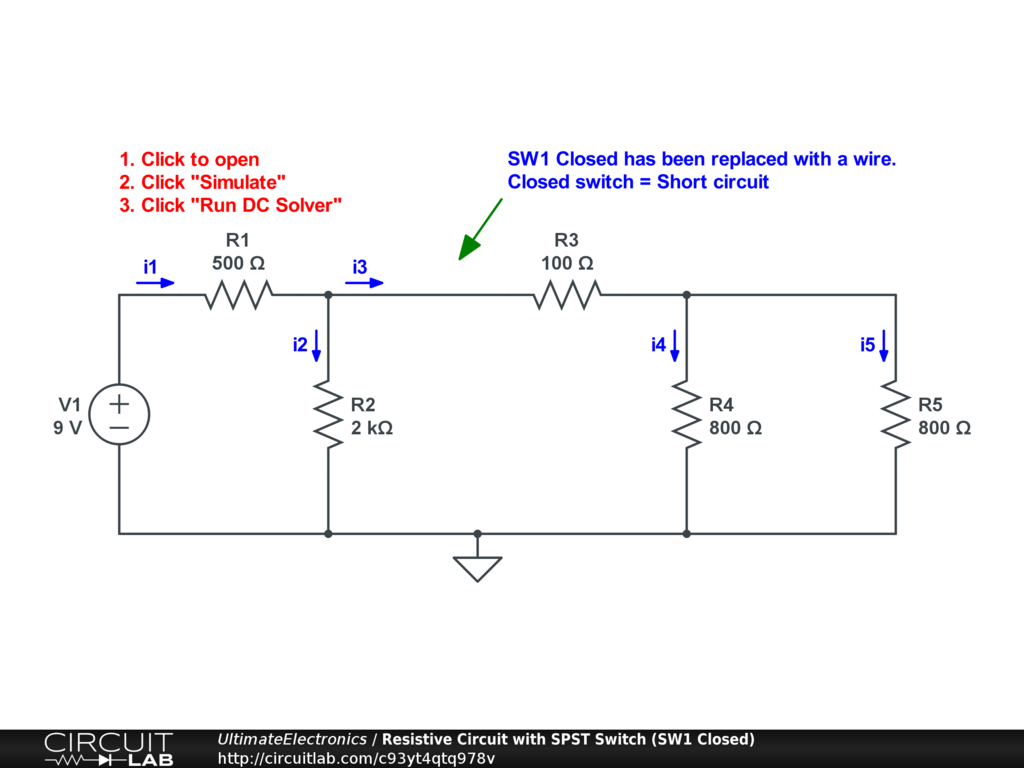From this schematic, we just have a jumble of five resistors in series and parallel. We can apply the combination rules carefully to get our effective resistance.

First, we can combine R4 and R5 in parallel. Since they have equal resistance, the parallel combination is just half of their individual resistance:

$$R_{\text{eq1}} = R_4 // R_5 = \frac{R_4 R_5} {R_4 + R_5} = \frac {800^2} {2 \cdot 800} = 400 \ \Omega$$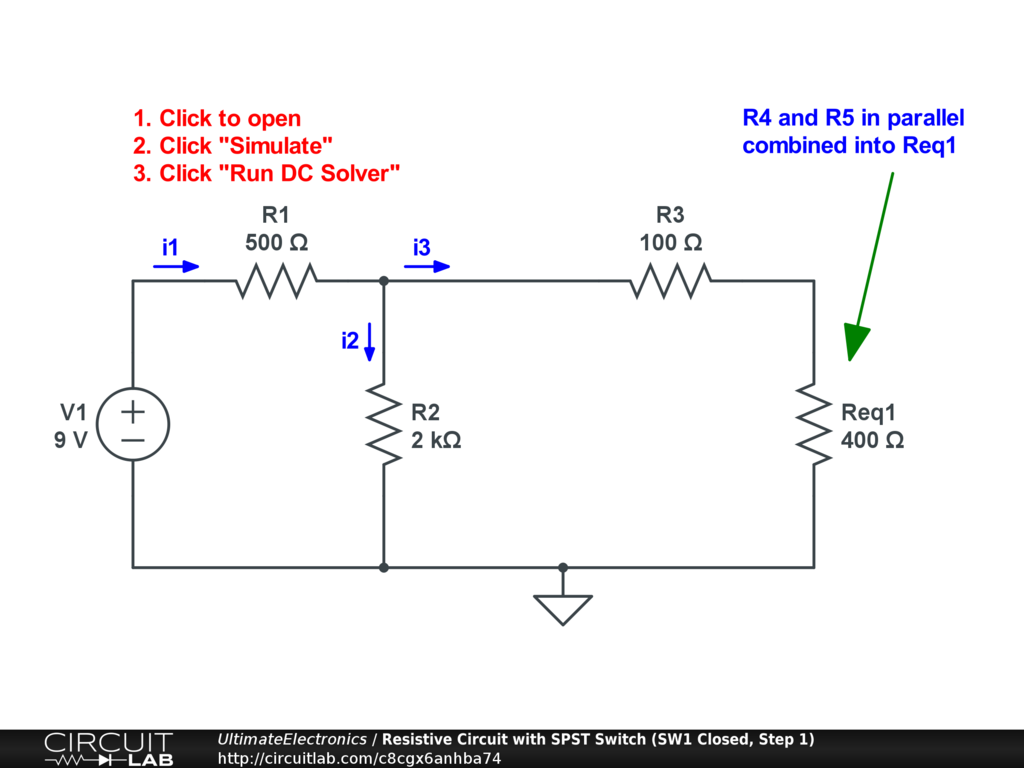Next, we can see that R3 is just in series with Req1, so we can add their resistances to get Req2:

$$R_{\text{eq2}} = R_3 + R_{\text{eq1}} = 100 + 400 = 500 \ \Omega$$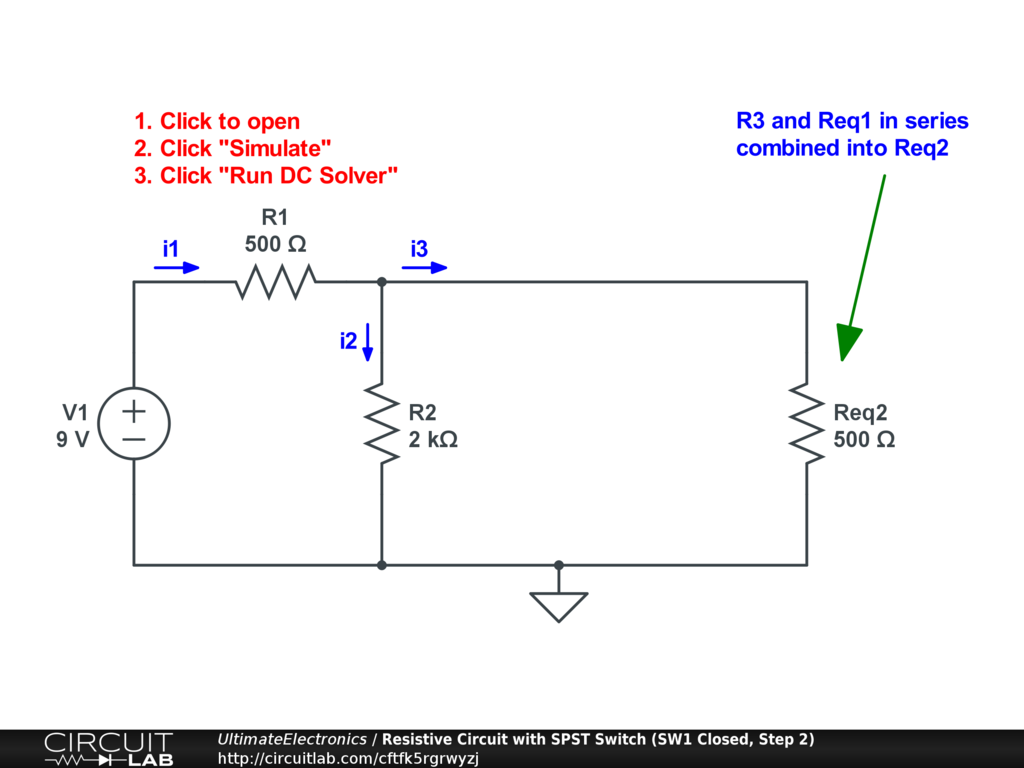Next, we combine the parallel R2 with Req2 to get Req3:

$$R_{\text{eq3}} = R_2 // R_{\text{eq2}} = \frac {2000 \cdot 500} {2000 + 500} = 400 \ \Omega$$

As a quick trick for solving parallel resistances like these by hand, notice that there's a fixed ratio between the 2000 and 500 values: a factor of 4. You can effectively think of the $500 \ \Omega$ resistor as being 4 parallel $2000 \ \Omega$ resistors, because $N$ same-size resistors in parallel will have a resistance of $R_{//} = \frac {R_x} {N}$. Then, you can think about the additional R2 as being just a 5th resistor in parallel with the other four! This means that the parallel combination is just like having $4+1 = 5$ of these $2000 \ \Omega$ resistors in parallel, for a total effective resistance of $\frac {2000} {5} = 400 \ \Omega$.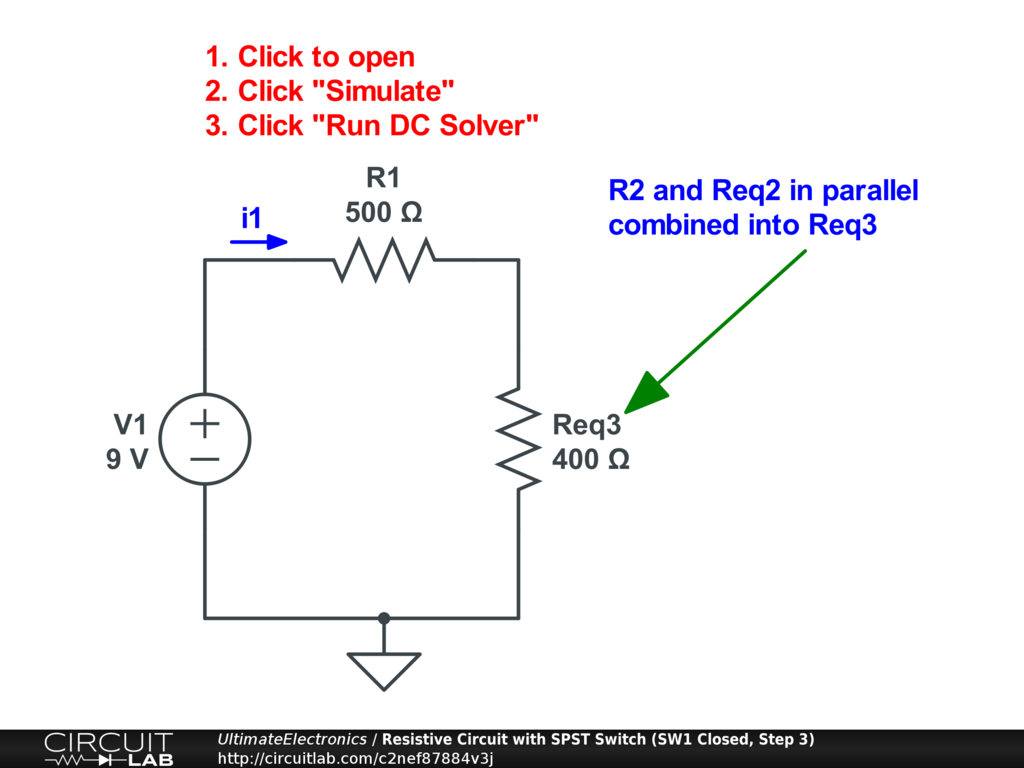Finally, we can combine the series R1 and Req3 to get Req4:

$$R_{\text{eq4}} = R_1 + R_{\text{eq3}} = 500 + 400 = 900 \ \Omega$$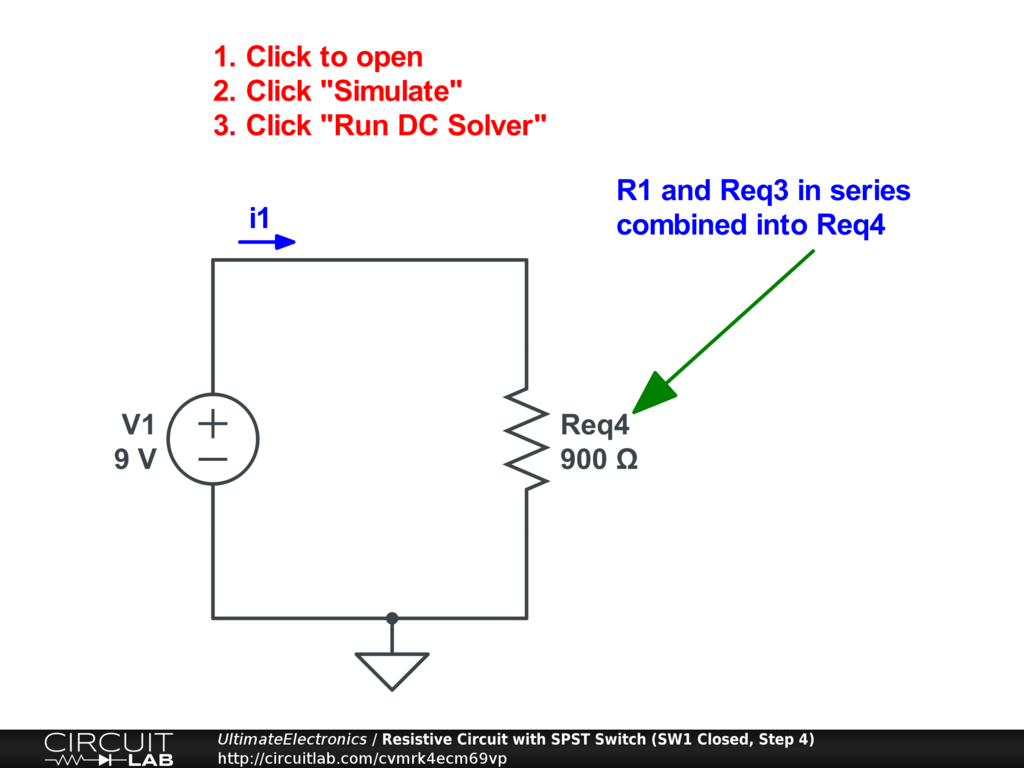From here, it's again easy to use Ohm's Law to find the total current:

$$i_1 = \frac {V} {R} = \frac {9} {900} = 0.01 = 10 \ \text{mA}$$

In this switch closed case, the other branch currents $i_2, i_3, i_4, i_5$ in the original circuit have their own values. But now that we have the total current, we can "unwind" our resistor simplifications and look at how the current divides through each branch.

If we scroll backwards through the resistor series-parallel simplifications, the first current split that we have to account for is between $i_2 \ \text{and} i_3$. We know that the total current is split:

$$10 \ \text{mA} = i_1 = i_2 + i_3$$

We also know a ratio of the total resistances of each path: $R_{\text{eq2}} = \frac {1} {4} R_2$. With this four-to-one resistance ratio, the path through Req2 will carry 4 times the current for the same voltage as R2. That means that Req2 will carry $\frac{4} {5}$ of the current, and R2 will carry $\frac {1} {5}$. Expressed as currents:

\begin{align} i_2 & = \frac {1} {5} i_1 = 2 \ \text{mA} && \text{(through R2)} \\ i_3 & = \frac {4} {5} i_1 = 8 \ \text{mA} && \text{(through Req2)} \\ \end{align}

Scrolling up further, the next split is when $i_3$ divides into $i_4$ and $i_5$. It happens that R4 and R5 have the same resistance, so the current divides equally between them:

\begin{align} i_4 & = \frac {1} {2} i_3 = 4 \ \text{mA} && \text{(through R4)} \\ i_5 & = \frac {1} {2} i_3 = 4 \ \text{mA} && \text{(through R5)} \\ \end{align}

We've now solved for all five branch currents, and could easily compute the node voltages. This example demonstrates how to use resistor series and parallel combination rules to solve resistive circuits quickly.

To quickly check our math, we can click the schematic below to open the original circuit in CircuitLab, double-click the switch and set it to be closed, and then run the DC solver to check the current values:Interactive Exercise Click to simulate the circuit after making switch SW1 closed.

Transient Switching Behavior

So far we've only talked about the steady state behavior of a circuit with switches in it. This applies if the circuit only has resistive elements. In the Lumped Element Model of an electric circuit, resistors have no memory and find a new equilibrium state instantaneously once a switch is flipped.

However, if our circuit has other components that do have any sort of memory or time-varying behavior, including capacitors or inductors, then we have a more complicated situation whenever a switch changes state.

In these cases, we have to consider two things:

1. Calculating the new steady state currents and voltages in the new switch configuration.
2. Calculating the transient behavior which includes intermediate, temporary voltages and currents that apply until the new steady state equilibrium is reached.

Consider this circuit with a switch that closes at time $t=10 \ \text{ms}$: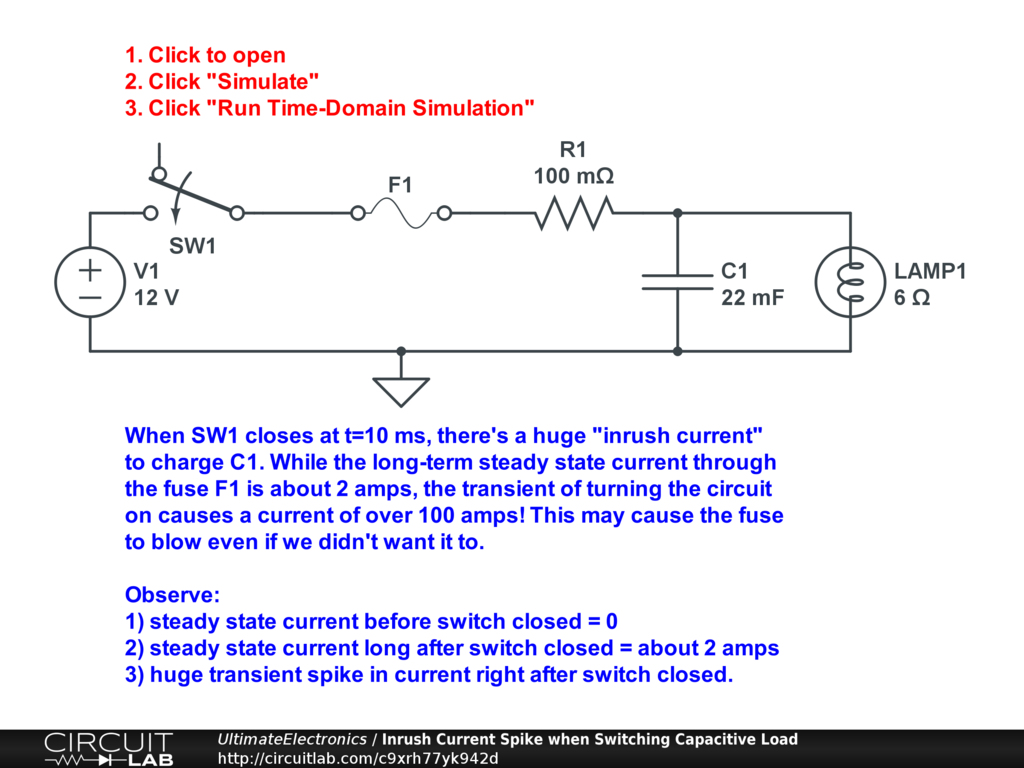Interactive Exercise Click the circuit and run the time-domain simulation. This is an example of a circuit that is switched between two different steady states, but has some interesting and important behavior as it's transitioning in between. Because of the capacitor C1, there's temporarily a huge current flowing right after the switch closes (peaking around 120 A), until the situation settles down to the new steady state current of just under 2 A.

The simulation software makes it easy to examine situations like these, but we can also gain intuition analytically. In the moment just after the switch closes, the capacitor C1 "looks like" a short circuit, causing an instantaneous peak current of about $I = \frac {V_1} {R_1} = \frac {12} {0.1} = 120 \ \text{A}$ to flow through the fuse and R1. In the long-term steady state, the capacitor carries no currrent at all, and so looks open-circuit, leaving a total current of $I = \frac {12} {6 + 0.1} \approx 1.97 \ \text{A}$ through the lamp. We'll talk more about capacitors in a later chapter.

In the steady-state-only world, with just ideal sources, resistors, and switches, if we had N two-position switches, we had to possibly calculate $2^N$ steady states of the circuit. However, once we allow transient behavior, the circuit may or may not fit into any of those steady states.

For example, consider this simple switching circuit with a switch SW1 that is being toggled open and closed four times per second: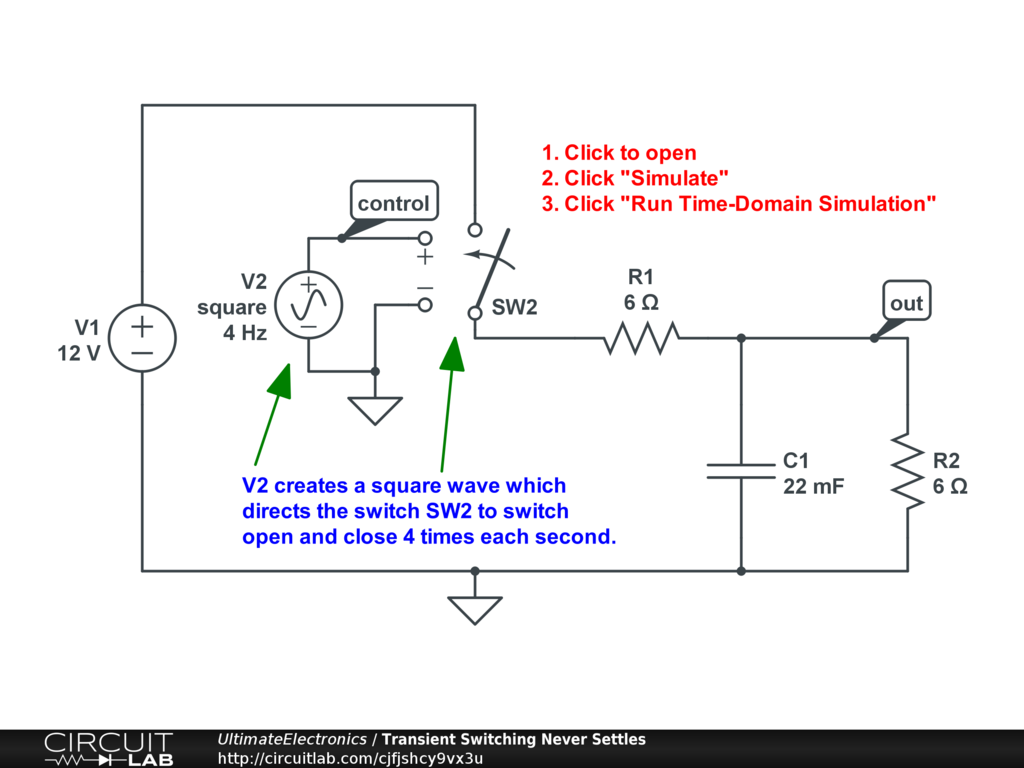Click the circuit and run the time-domain simulation. As is, the circuit never has enough time to settle down to either of its steady states. Instead, it's always in motion toward one or the other.

What happens if we change the frequency at which we're toggling the switch? Double click on the voltage function generator V2 and change the frequency to be 1 Hz instead of 4 Hz, and re-run the time-domain simulation. Now, the output voltage just about reaches its steady state at around $V_{\text{out}} = 6 \ \text{V}$ when the switch is closed (i.e. when $V_{\text{control}} = 5$).

What happens if we change the value of the capacitor C1? Double click C1 and change it to be "1m" instead of "22m", and re-run the time-domain simulation. Now, the system reaches its new steady state very quickly after each transition of the switch.

Analytically, this example has two easy-to-solve steady states. (Note that at steady state, we can consider capacitors to be open circuit as though they were removed entirely from the circuit. We'll address this in more detail in a later chapter.) When the switch is open, no current flows through R1 or R2, so $V_{\text{out}} = 0$. When the switch is closed, R1 and R2 form a simple voltage divider with two equal resistances, so $V_{\text{out}} = \frac {1} {2} V_1 = 6 \ \text{V}$.

The transient behavior is slighty more involved to describe. We'll later talk about time constants for RC circuits. In this case, when charging, the time constant is:

$$\tau_1 = (R_1 // R_2) C_1 = (3 \ \Omega) (0.022 \ \text{F}) = 0.066 \ \text{s}$$

When discharging, R1 is disconnected, and the time constant is slightly longer:

$$\tau_2 = R_2 C_1 = (6 \ \Omega) (0.022 \ \text{F}) = 0.132 \ \text{s}$$

Our switch is toggling open and closed at a frequency of 4 Hz, meaning it goes through a full cycle every 0.250 seconds. It's in each state for half that time, or 0.125 seconds. Since the time RC time constants are roughly similar in duration to the switching period, the circuit has time to make some progress toward its steady-state goal, but will not get all the way there.

In contrast, if we make the RC time constants much shorter than the switching period $\tau_{\text{RC}} \ll \tau_{\text{switching}}$, for example by changing from a 22 mF capacitor to a 1 mF one as described above, then the circuit will have time to reach its final value.

What happens if we instead make the switching period significantly shorter than the RC time constant, $\tau_{\text{RC}} \gg \tau_{\text{switching}}$? What happens to the "sawtooth" ripple shape of $V_{\text{out}}$? Simulate and find out.

We'll talk much more about time constants and RC circuits in later chapters.

Simulating Buttons & Switches

In a circuit simulation environment, we need to think carefully about what exactly we want a switch to do in the context of our simulation.

Some "toy" simulators will allow for interactive clicking of buttons and switches during a simulation, but beyond the very earliest learning stages, these interactive simulations have no real-world applicability. Simulations should be designed for controlled repeatability, where we can understand the effects of making changes in our circuit, which calls for a repeatable way of controlling switches.

Time-Controlled Switch

The simplest switch is a time-controlled switch, which is modeled as an SPDT switch that flips from one state to another at a pre-specified time. The trigger time can be set by double-clicking the switch.

We've already seen an example of a time-controlled switch in a circuit simulation earlier in this section:Interactive Exercise Click the circuit to open it, and then double-click on the switch SW1. Here, you can see that it's set to change at time "10m", which represents $t = 10\ \text{ms}$. Try changing the switching time and then re-run the circuit simulation.

Time-controlled switches are the easiest to understand when doing time-domain simulations. Many classwork problems involving RC or RL circuits involve asking what happens when a switch is closed or opened at a particular time, and the time-controlled switch provides an easy way to model this directly.

Note that you should be careful when defining a switch to change states at exactly $t=0$. In most cases, the simulator will automatically "do the right thing," and start out with the pre-flipped state for the very first datapoint, and then toggle switch immediately after $t=0$. However, if you're not sure, change the trigger time to be some small positive amount so you simulate the original state of the circuit first.

"Skip Initial" Simulation Mode

Normally, when any circuit simulation software runs a time-domain simulation, it first finds the initial steady-state solution for the system before $t=0$.

In a circuit like this one: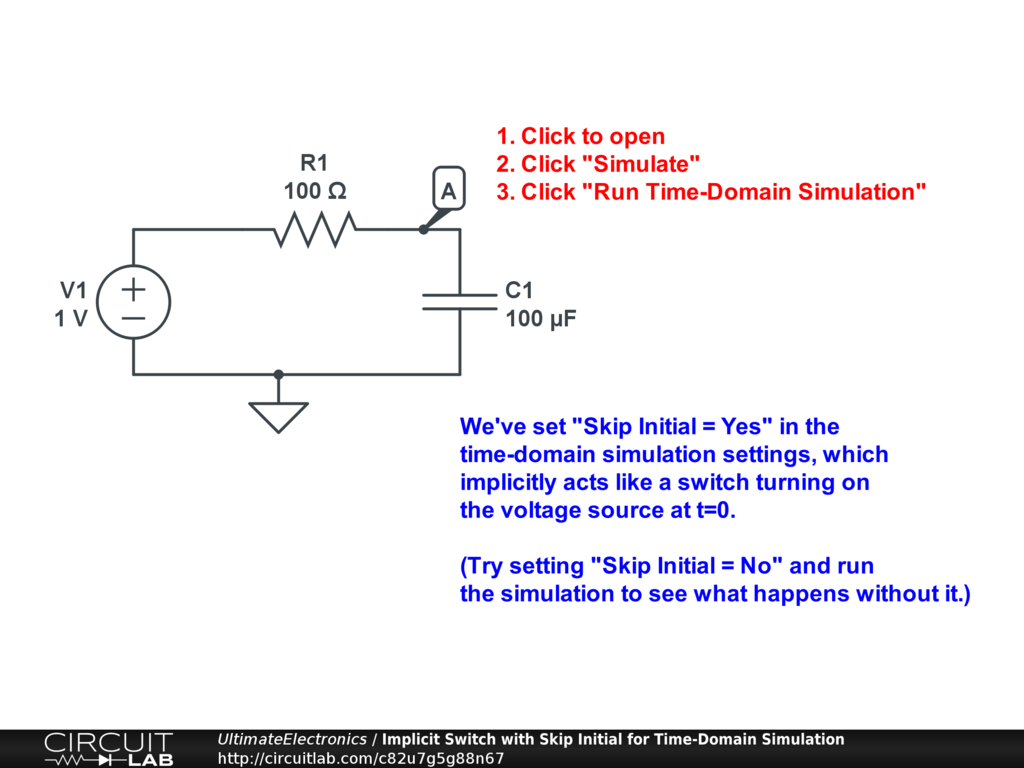Interactive Exercise Click to simulate the circuit above.

This initial steady-state solution will treat the capacitor as being already charged to its long-term DC value, $V_A = 1 \ \text{V}$. When we run the time-domain simulation, we'd then get a boring flat line, because nothing is changing!

Instead, we've set "Skip Initial = Yes" inside the time-domain simulation settings. This tells the simulator to entirely skip the process of solving for the initial state of the circuit before $t=0$, so instead, the capacitor defaults to being totally uncharged.

Click the circuit and run the simulation with "Skip Initial = Yes" to confirm that the capacitor now starts off uncharged, and then charges up towards its final value. Now, change it to "Skip Initial = No" (the default for CircuitLab) and see what happens.

While this setting "works" for simple RC circuits and the like, it tends to create issues with more complicated circuits, like those containing transistors or op-amps, because they have internal state (like internal capacitors) that won't be charged to the correct starting values. Instead, we highly recommend simply using a time-domain switch set for $t=0$ to be completely explicit about the switching start-up of your circuit, rather than relying on this simulator setting.

Voltage-Controlled Switch

The voltage-controlled switch is one of the most powerful elements in simulation. (In practice, transistors and relays can act like voltage-controlled switches, but here we're just talking about theory and simulation.) It changes between open and closed depending on the voltage difference at its control terminals.

Below are a few different examples of how to use a voltage-controlled switch. Click each, run the simulation, and then try to modify and understand how it works.

1) In an example we already explored earlier in this section, we can use a voltage function generator set to produce a square wave output to produce a control signal for a voltage-controlled switch. Note that we've set the amplitude and offset of the function generator V2 to match the transition voltage point of the switch SW2:Interactive Exercise Click to simulate the circuit above.

2) Our control function can be a simple function of time, such as $V_{\text{CONTROL}} = 5 (T > 3)$. This function evaluates to 0 for times before $t=3$, and evaluates to 5 for times after: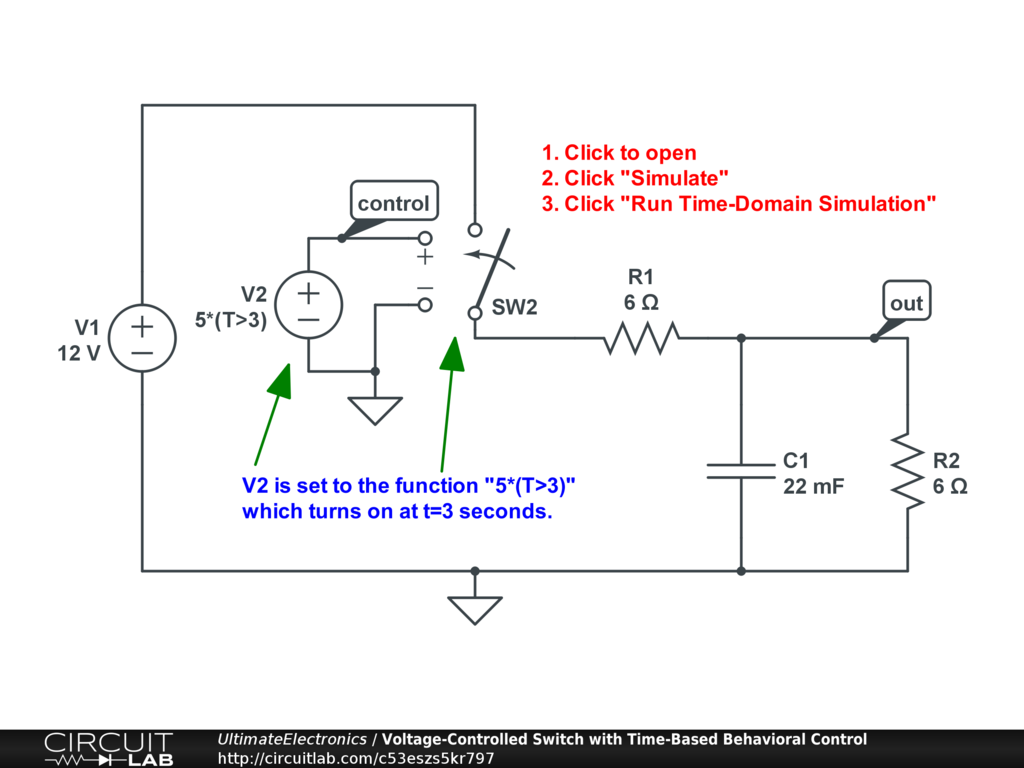Interactive Exercise Click to simulate the circuit above.

3) Our control can be a piecewise-step function, such as $V_{\text{CONTROL}} = \text{PWS}(0,0,0.9,5,1,0)$. The PWS function takes a list of $(t_i,x_i)$ pairs, so at $t=0$ the function will stay at $V=0$, until $t=0.9$ at which time $V=5$, and then at $t=1$ the control voltage will go back to zero. In this way, we can create arbitrarily complicated signals: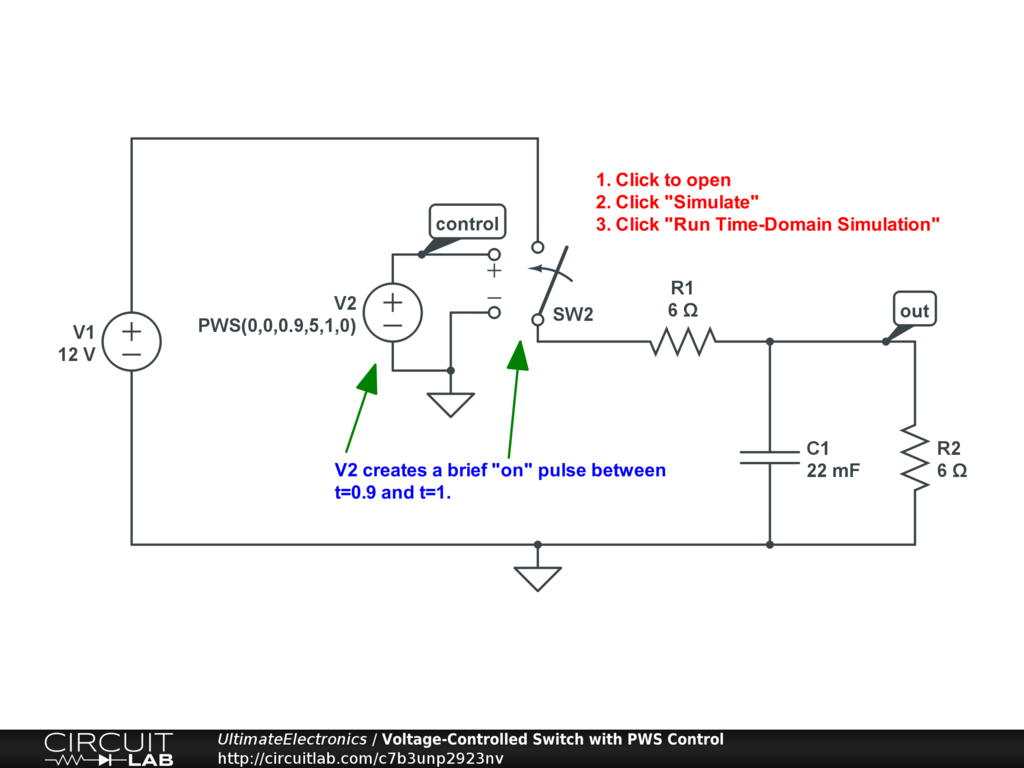Interactive Exercise Click to simulate the circuit above.

4) Instead of specifying our time-value pairs in the PWS function, we can also specify them as a CSV file. Double-click the CSV source V2 to see inside: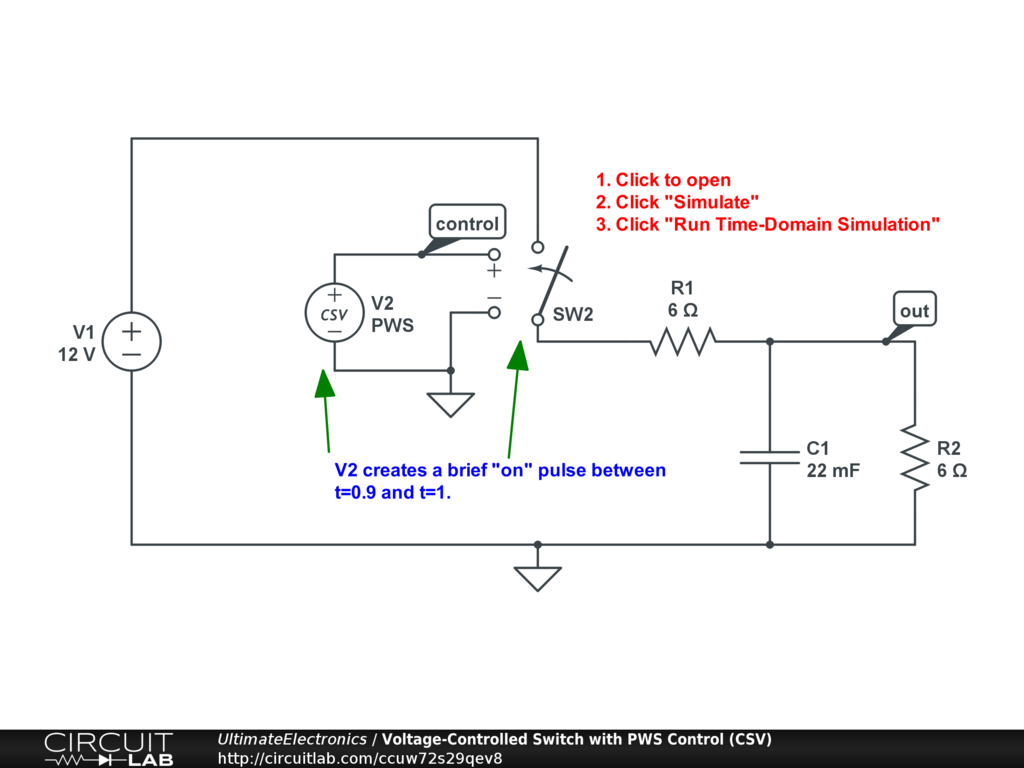Interactive Exercise Click to simulate the circuit above.

5) Instead of PWS, we can use PWSREPEAT, which keeps repeating the same pattern over and over again: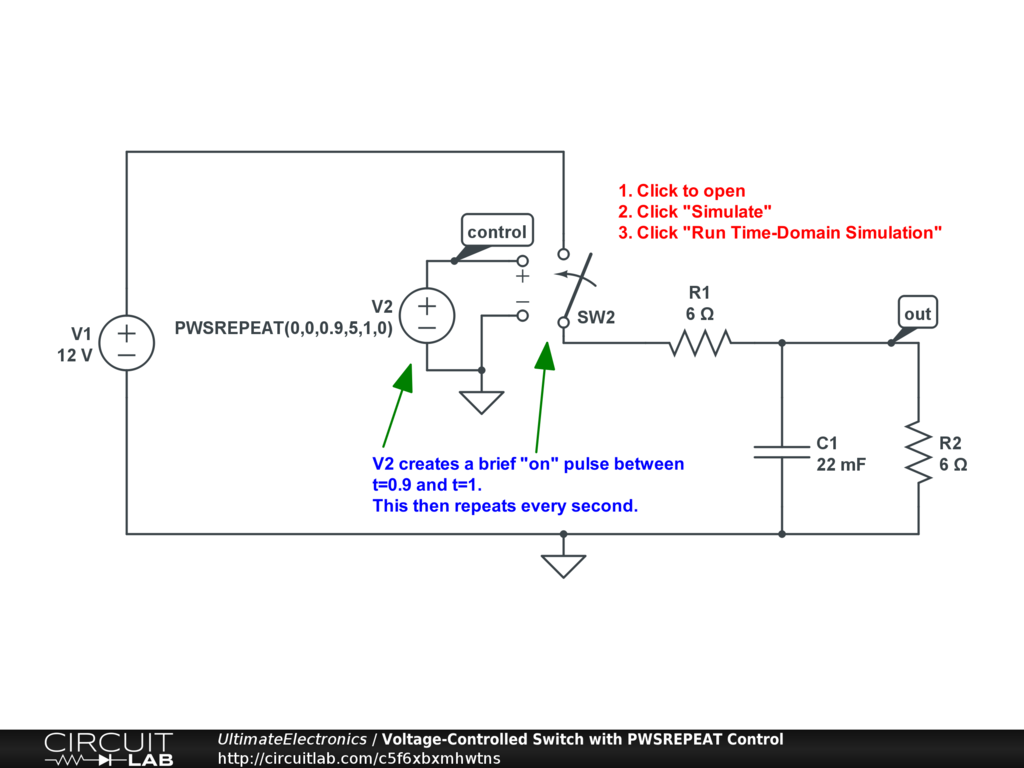Interactive Exercise Click to simulate the circuit above.

6) We can also use a digital clock source to control a voltage-controlled switch: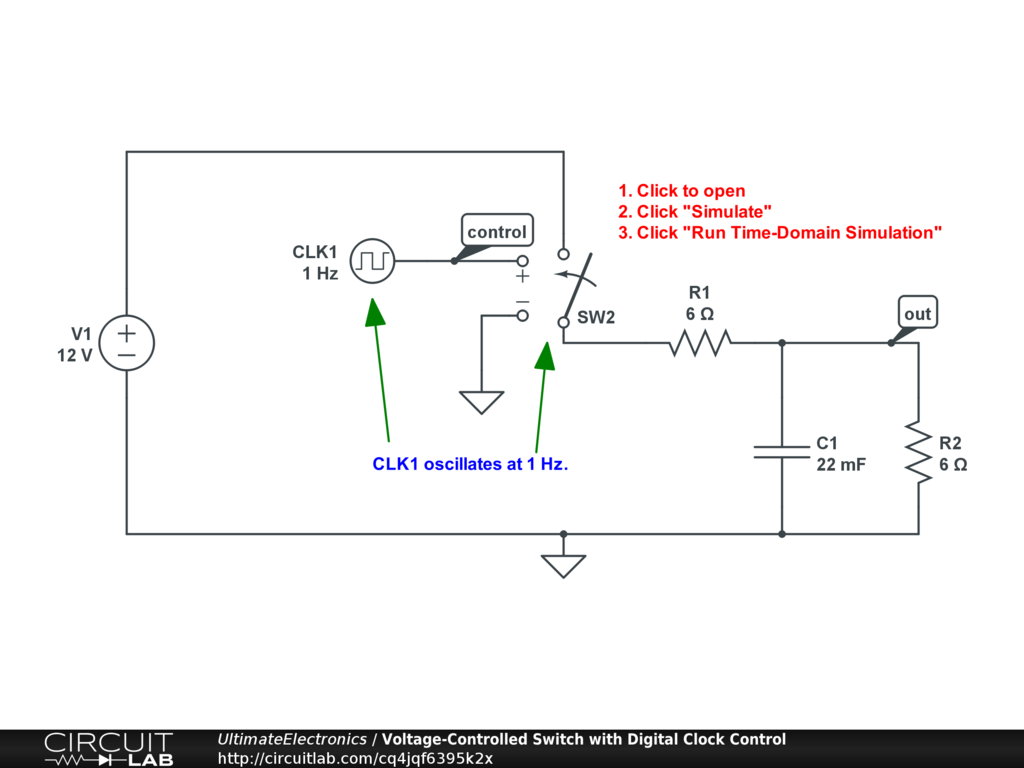Interactive Exercise Click to simulate the circuit above.

7) Finally, we can use a voltage within the circuit itself as the trigger. This example is a bit more complicated, but it uses the fact that the voltage-controlled switch model has hysteresis. Hysteresis means that after the switch changes from one state to the other, there's some memory so that a larger wiggle is required to get it to switch back to the first state. This is configured within the V_H parameter of the switch SW2. Double-click SW2, try changing the hysteresis voltage V_H, and re-run the simulation: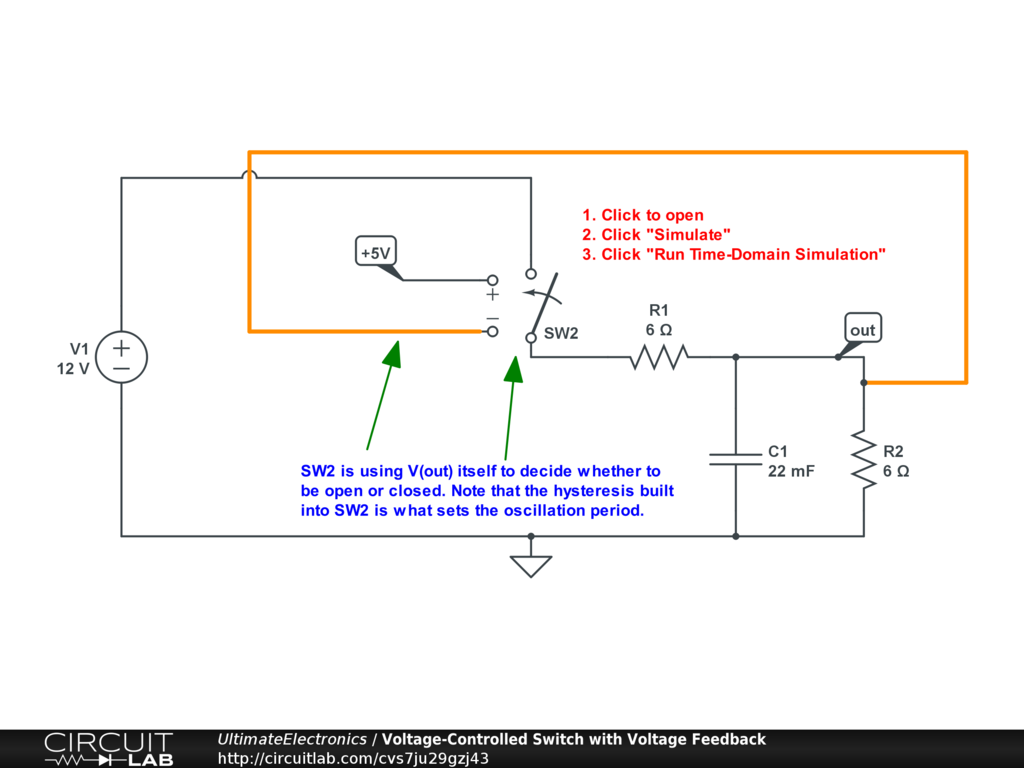Interactive Exercise Click to simulate the circuit above.

What's Next

In the next section, Voltage Dividers, we'll look at a common arrangement of resistors in series that come up extremely frequently in circuit design and analysis.